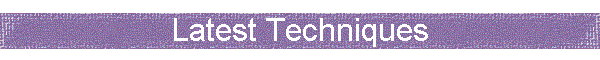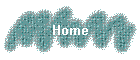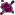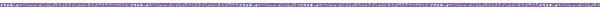Related 'Python for Delphi' Links on this site:tutorial - Andy's Python Delphi Tutorial - Getting started with the basics.  Also use these techniques if you are using Delphi 5 or below, and Python 2.0 or below.   Includes info on getting these free components.code - Here are Andy's Extensions  - a delphi unit that adds a few utility functions to Python for Delphi.tutorial - Here is a later tutorial using the Latest Techniques in Python for Delphi - use these if you have Delphi 6 and Python 2.1 or higher.discussion & tips - Here is a discussion and tips on python for delphi deployment issues.animated slideshow tutorial  - Here is an animated visual viewlet demo of using the Python for Delphi components to build a Delphi app that talks to python.example and screenshot of a Delphi GUI application which uses python code for all its business logic.Latest Python Delphi techniques & developments

As of October 2001 a totally new way of talking to python we developed, within the Python for Delphi framework.  You use variants, and get smart references to python objects.

So whilst before you could create a reference to an instance to a class, in an olevariant.  Now we use variants.

Also we can instantiate classes directly (without needing to use my PyClass() function etc. etc.

Here is what Morgan, one of the authors of the components has to say:
http://groups.yahoo.com/group/pythonfordelphi/message/210The latest way of programming in python for Delphi if you have Delphi 6 and python 2.1 or higher.

-Andy Bulka.

These techniques are demonstrated in Demo25 in the examples folder of your Python for Delphi distribution.

The old vs. the new ways.

Because Delphi 6 has custom variants, they can point to specific smart proxies for python objects.  Before Delphi 6, you could have an oleVariant pointing to a python instance, but you couldn't do the smart things like know that it was a list type etc.

The following examples assume you have a module called LoginMgr.py in the python path or in the same folder as your application  .exe that you have built in Delphi.  Note that the python file LoginMgr.py contains within it a python class called LoginMgr which has a method called somemethod.

 Old way   (see my basic tutorial for more info  on the AndyDelphiPy functions.) New way  (requires Delphi 6) // Drop a TPythonAtomEngine onto your form  // or datamodule and name it PE // You also need to drop a pythonDelphiVar  // component and call it pdv uses AndyDelphiPy; var   obj : olevariant; begin AndyDelphiPy.PyExeFile('LoginMgr.py', PE); obj  := AndyDelphiPy.PyClass('LoginMgr()', pdv, PE); obj.somemethod()    // call the method // Drop a TPythonAtomEngine or TPythonEngine  // onto your form or datamodule.   uses VarPyth; var   mymodule, obj: variant; begin mymodule := Import('LoginMgr'); obj := mymodule.LoginMgr(); obj.somemethod()    // call the method

Note that it it possible to slightly mix the old and new way, so that if you use the AndyDelphiPy.PyExeFile('LoginMgr.py', PE); to import the module then you can then switch to the new way, declare an obj: variant; then instantiate an instance using obj := MainModule.LoginMgr();  However you still need Delphi 6 and so you might as well just use the new way properly.

Widestrings

Declare your delphi strings widestrings if you want to get more than 255 chars back from calls to python methods that return strings. e.g.

var
s : widestring;
begin
s := mypythonclassinstance.somemethod() ;
showmessage(s) ;

Booleans

If your python method call returns 1 or 0 and this is supposed to be interpreted as a boolean, then cast it inside Delphi e.g.

if Boolean( mypythonclassinstance.somemethod()) then
....

Accessing syspath directly

Here is a function that accesses a global variable called SysModule and access the syspath directly.

This function also calls VarIsPythonSequence which tests to see if the parameter passed is a list or not.

procedure TForm1.Button2Click(Sender: TObject);
const
LIB = 'E:\\ZopeWebSite\\bin\\lib';
LIBDLL = 'E:\\ZopeWebSite\\bin\\DLLs';
var
re : variant;
m : variant;
begin
Assert(VarIsPythonSequence(SysModule.path));
displaySysPath(ListBox1);
if not Boolean(SysModule.path.Contains(LIB)) then
SysModule.path.insert(0,LIB);
SysModule.path.append(LIBDLL);
memo1.lines.Add('SysModule.path now is ' + \
SysModule.path + #13#13);
displaySysPath(ListBox1);
fixSysPath;
re := Import('re');
showmessage(re);
m := Import('xml.dom.minidom');
showmessage(m);
end;

Playing with sys paths

This is an example of how to set the python system path as seen by delphi's instance of the python interpreter (as represented by the pythonEngine component).  Note that it is imperative that you have \\ as the slashed in your path as otherwise things like \fred will actually be interpreted as \f (whatever that escaped character is) plus 'red'.

Technique 1

procedure TForm1.fixSysPath;
const
LIB = 'E:\\ZopeWebSite\bin\\lib';
LIBDLL = 'E:\\ZopeWebSite\\bin\\DLLs';
begin
// this is illegal
// SysModule.path.Clear;
// this will work with latest python for delphi components OK.
//SysModule.path := NewPythonList;
// this is a boring but effective solution as well.
while SysModule.path.Length > 1 do
SysModule.path.pop;
SysModule.path.append(LIBDLL);
SysModule.path.append(LIB);
end;

Technique 2

procedure TForm1.btnClearSyspathToJustLibClick(Sender: TObject);
var
currdir, libdir : string;
begin
currdir := ExtractFilePath( Application.ExeName );

NOTE: Simply putting a window path as the currdir will ultimately fail since the paths returned by Delphi have single slashes and python needs wither unix slashes or \\ slashes. See here for an algorithm to handle this.

libdir := EnsurePathHasDoubleSlashes(libdir);
libdir := currdir + 'Lib';
SysModule.path := NewPythonList;
// Relies on Jan 2002 install of python for Delphi components
SysModule.path.append(currdir);
SysModule.path.append(libdir);
end;

NOTE:  See the python for delphi deployment section for a more in-depth discussion of paths.

Supplimentary utility to display the python syspath in a delphi gui control.

procedure TForm1.btnDisplaySysPathClick(Sender: TObject);
begin
ListBox1.clear;
displaySysPath(ListBox1);
end;

Writing a Delphi function that uses a python function to do the hard work.

Here is an example of writing a delphi utility function that takes a string, and splits it up (delimited by comma) and puts the result into a delphi list box.  We are using python split function to do the splitting - cool eh?

procedure TForm1.splitAstring(str:string; lstbox: TListBox);
var
s, lzt : variant;
i : integer;
begin
s := VarPythonCreate(str);
// convert normal string into a python string.
lzt := s.split(',');
for i := 0 to lzt.Length-1 do
end;

Displaying the python syspath in a delphi listbox

Even though we have a pointer to a python list object (via a Delphi variant), we still have to call .GetItem(i) on a python list rather than the python syntax of lzt[i] - why?  Because we are in Delphi and thus we cannot use python syntax.

procedure TForm1.displaySysPath(lstbox: TListBox);
var
lzt : variant;
i : integer;
begin
Assert(VarIsPythonSequence(SysModule.path));
lzt := SysModule.path;
for i := 0 to lzt.Length-1 do
end;

var
m, doc, top : variant;
s : string;
begin
fixSysPath;
displaySysPath(ListBox1);
m := Import('base64');
showmessage(m);
s := m.encodestring('this is some text which I am going to encode then decode again.');
showmessage(s + #13+#13 + m.decodestring(s));
m := Import('xml.dom.minidom');
doc := m.Document();
showmessage(doc);

top := doc.createElement( 'Workspace' );
top.setAttribute('Version', '1.1 beta4');
doc.appendChild(top);
s := doc.toxml();
showmessage('doc.toxml()' + #13+#13 + s);
end;

Ensure you have a TPythonAtomEngine or TPythonEngine onto your form or datamodule.

var
mymodule, obj: variant;
begin
obj.somemethod()    // call the methodMorgan's original tutorial on the new techniques

VarPyth unit:

It wraps a Python object and lets you act on it (call methods or use properties) like you would do in Python, and like you did with PythonAtom, but there are differences:

the variant always maintains its link to a Python object, and is never casted to a basic variant type (integer, string, array) when returning a value, like it was with PythonAtom.

v1 := VarPythonCreate(1);
v2 := VarPythonCreate(2);

v1 + v2 will return a new variant that will hold the a Python object that is the result of the addition, and the addition will be performed by Python itself, using the PyNumber_Add API.

Previously, with PythonAtom, if you accessed a property or called a method, the value returned by Python was automatically converted to a basic variant type, or wrapped up into an PythonAtom variant otherwise.

The main advantage of this solution was to convert Python sequences (lists or tuples) into array of variants, letting us access them using the index operator (list[x]).

Now, it is not possible anymore, as the returned sequence will be returned as a new custom variant, and our custom variant doesn't support indexing!!!  I don't know how to do it? Maybe adding the flag varArray to our VType and setting array bounds that let us access any item, but I'm not sure... Anyway, it could work with sequences, but not with dictionaries, as it
accepts any type as a key.

So, to work around this problem I added several special methods/properties:

foo.GetItem(index): same as foo[index]
foo.SetItem(index, value): same as foo[index] = value
foo.Length or foo.Length(): same as len(foo)
foo.GetSlice(index1, index2): same as foo[index1:index2]
foo.SetSlice(index1, index2, value): same as foo[index1:index2] = value
foo.Contains(value): same as value in foo
foo.DeleteItem(Index): same del foo[index]

Note that my special methods are not case sensitive.

Note also that you must use the parenthesis with functions or methods that have no argument, to distinguish your call with a property access:

list.sort()

if you write list.sort only, you'll get the instance method object instead of performing the sort action.

The advantage of the new solution is that you always work with the Python objects, and you can do anything you want with them, like you would in Python. The arithmetic operations, or comparisons, work the same, and you can't add an integer to a string, for instance, as you can with variants.

v1 := VarPythonCreate(1);
v2 := v1 + 'hello'; --> Python exception

but

v2 := 'hello' + v1;

will work as the string is a variant that forces the right operand to be casted to a string variant, letting the concatenation to apply.      But

v2 := VarPythonCreate('hello') + v1; --> Python exception

And

v2 := v1 + 'hello';

--> Python exception as hello is converted to a Python variant and then an addition is beformed between a number and a
string, and Python does not like it!

You can write also:

v1 := VarPythonCreate([1, 2, 3]);
v2 := v1 + VarPythonCreate([4, 5, 6]); --> you'll get: [1, 2, 3, 4, 5, 6]

There are several facility functions that let you test the different types of Python objects, or that let you import other Python modules, or access the None object.

Now, you can easily execute a Python script and then access the global vars of the main module:

GetPythonEngine.ExecString('x = 2');
ShowMessage( MainModule.x ); // will display 2

you can access the sys module with the function SysModule, or you can import any module with the Import function, that returns the imported module object.

myModule := Import('myModule');
myModule.foo(bar);

You can create a Python variant with:

v := VarPythonCreate(myPythonObjectPointer);
v := VarPythonCreate(1);
v := VarPythonCreate('abc');
v := VarPythonCreate(3.14);
v := VarPythonCreate(True);
v := VarPythonCreate(VarArrayOf([1, 2, 3]));
v := VarPythonCreate([1, 2, 3]);
v := VarPythonCreate([1, 2, 3], stTuple);
v := None;
v := NewPythonList;
v := NewPythonList(3);
v := NewPythonDict;

you can access to the Python object pointer stored in the variant with

ptr := ExtractPythonObjectFrom(v);

you can test the variant with:

VarIsPython(v)
VarIsSequence(v)
VarIsMapping(v)
VarIsNumber(v)
...

you can check if 2 variants shares the same Python object with

VarIsSame(v1, v2)

you can check if an instance has a class that is or inherits from a class with:

VarIsInstanceOf(AInstance, AClass)

or you can check the inheritence between 2 classes with

Note that these 2 functions requires Python 2 or later.

You can check None with:

VarIsNone(v)
or
v = None
or
VarIsSame(v, None)

You can instanciate a class like you would in Python:

v := MainModule.MyClass()

Note, that your class object must the property of a container and can't be stored in a variant:

cl := MainModule.MyClass; // cl refers to MyClass
v := cl(); // won't work because Delphi refuses to compile it!

You can cast a Python variant to another type any time simply by assigning it to a variable of the required type or by forcing the cast with:

if Boolean(list.Contains(1)) then ...

MorganDemo25

Here are some choice extracts from demo25 (from the Demo25 folder that you get when you download the Python for Delphi components), which show how to use this stuff:

Playing with lists and dictionaries

procedure TMain.btnTestSequencesClick(Sender: TObject);
var
a, b, c : Variant;
begin
// initialize the operands
// you can either use the overloaded function with an array of const
// or use the VarArrayOf function that returns an array of variants that will
// be casted to a Python list.
a := VarPythonCreate([1, 2, 3]);
Assert(VarIsPython(a));
Assert(VarIsPythonSequence(a));
Assert(VarIsPythonList(a));
Assert(a.Length = 3); // this is a special property that does the same as: len(a) in Python
Assert(a.Length() = 3); // this is a special method that does the same as the special property
Assert(len(a) = 3);
Assert(a.GetItem(0) = 1); // this is a special method that lets you do the same as: a in Python
Assert(a.GetItem(1) = 2);
Assert(a.GetItem(2) = 3);
Assert(String(a) = '[1, 2, 3]');
b := VarPythonCreate(VarArrayOf([4, 5, 6]));
Assert(VarIsPython(b));
Assert(VarIsPythonSequence(b));
Assert(VarIsPythonList(b));
Assert(b.Length = 3);
Assert(b.Length() = 3);
Assert(len(b) = 3);
Assert(b.GetItem(0) = 4);
Assert(b.GetItem(1) = 5);
Assert(b.GetItem(2) = 6);
Assert(String(b) = '[4, 5, 6]');
// concatenation
c := a + b;
// check result of operation
Assert(String(c) = '[1, 2, 3, 4, 5, 6]');
// check that operation did not change the content of operands.
Assert(String(a) = '[1, 2, 3]');
Assert(String(b) = '[4, 5, 6]');
// now with a litteral: note that with D6 SP1, we can't
// concatenate a custom variant with an var array of variants
c := a + b + VarPythonCreate(['Hello', 'World!', 3.14]);
Assert( String(c) = '[1, 2, 3, 4, 5, 6, ''Hello'', ''World!'', 3.1400000000000001]' );
c := a + VarPythonCreate(['Hello', 'World!', 3.14]) + b;
Assert( String(c) = '[1, 2, 3, ''Hello'', ''World!'', 3.1400000000000001, 4, 5, 6]' );
c := VarPythonCreate(['Hello', 'World!', 3.14]) + a + b;
Assert( String(c) = '[''Hello'', ''World!'', 3.1400000000000001, 1, 2, 3, 4, 5, 6]' );
// multiplication
c := a * 3; // in Python the multiplication of sequence concatenates n times the sequence
Assert( String(c) = '[1, 2, 3, 1, 2, 3, 1, 2, 3]' );
// comparisons
//------------
// equal
c := a = b;
Assert(c = False);
c := a = a;
Assert(c = True);
Assert( String(a) = '[1, 2, 3]');
// not equal
c := a <> b;
Assert(c = True);
Assert( not (c = b) );
c := a <> a;
Assert(c = False);
Assert( String(a) = '[1, 2, 3]');
// greater than
c := a > b; Assert(c = False);
c := b > a; Assert(c = True);
Assert( String(a) > '[1, 1, 1]');
// greater or equal than
c := a >= b; Assert(c = False);
c := b >= a; Assert(c = True);
c := a >= a; Assert(c = True);
Assert( String(a) >= '[1, 2, 3]' );
// less than
c := a < b; Assert(c = True);
c := b < a; Assert(c = False);
Assert( String(a) < '[4, 4, 4]');
// less or equal than
c := a <= b; Assert(c = True);
c := b <= a; Assert(c = False);
c := a <= a; Assert(c = True);
Assert( String(a) <= '[1, 2, 3]');
// copy
c := a;
Assert( c = a);
Assert( VarIsSame(c, a) ); // checks if 2 variants share the same Python object.
// sequence methods:
c := b + a;
c.sort(); // note that you must you the parenthesis to distinguish the
// call between a method or a property.
Assert( c = (a+b) );
c := NewPythonList; // facility for building sequences
Assert( not VarIsTrue(c) ); // c is false because it's an empty collection
c.append(1);
c.append(2);
c.append(3);
Assert( VarIsTrue(c) ); // c is true because it's not an empty collection
Assert(c = a);
Assert( c.pop() = 3 );
Assert( String(c) = '[1, 2]');
c := NewPythonList(3); // facility for building sequences
c.SetItem(0, 1);
c.SetItem(1, 2);
c.SetItem(2, 3);
Assert(c = a);
c.DeleteItem(1);
Assert(c = VarPythonCreate([1,3]));
Assert(VarPythonCreate([1,2,3,4]).GetSlice(1, 3) = VarPythonCreate([2,3]));
// same as x = [1,2,3,4]; x[1:3]
Assert(VarPythonCreate([1,2,3,4]).GetSlice(1, Ellipsis) = VarPythonCreate([2,3,4]));
// same as x = [1,2,3,4]; x[1:]
Assert(VarPythonCreate([1,2,3,4]).GetSlice(1, -1) = VarPythonCreate([2,3]));
// same as x = [1,2,3,4]; x[1:-1]
c := VarPythonCreate([1,2,3,4]);
c.SetSlice(1, 3, VarPythonCreate([7, 8, 9]));
Assert( c = VarPythonCreate([1, 7, 8, 9, 4]) );
Assert( Boolean(c.Contains( 7 )) ); // same as 7 in c
Assert( not Boolean(c.Contains( 77 )) );
c.DelSlice(1,3);
Assert( c = VarPythonCreate([1,9,4]) );
c := VarPythonCreate([1, 2, 3, 4], stTuple); // test a tuple
Assert( VarIsPythonTuple(c) );
Assert( VarIsPythonSequence(c) );
Assert( c.GetItem(1) = 2 );
Assert( c.Length = 4 );
c := NewPythonTuple(3);
c.SetItem(0, 1);
c.SetItem(1, 2);
c.SetItem(2, 3);
Assert( VarIsPythonTuple(c) );
Assert( VarIsPythonSequence(c) );
Assert( c.GetItem(1) = 2 );
Assert( c.Length = 3 );
// Done!
Log('Sequence test was Ok.');
end;
procedure TMain.btnTestMappingsClick(Sender: TObject);
var
a, b, c, keys, values : Variant;
begin
// initialize the operands
a := NewPythonDict;
Assert(VarIsPython(a));
Assert(VarIsPythonMapping(a));
Assert(VarIsPythonDict(a));
a.SetItem( 'a', 1 );
a.SetItem( 'b', 2 );
a.SetItem( 'c', 3 );
Assert(a.Length = 3);
// this is a special property that does the same as: len(a) in Python
Assert(a.Length() = 3);
// this is a special method that does the same as the special property
Assert(len(a) = 3);
Assert(a.GetItem('a') = 1);
// this is a special method that lets you do the same as: a in Python
Assert(a.GetItem('b') = 2);
Assert(a.GetItem('c') = 3);
b := NewPythonDict;
Assert(VarIsPython(b));
Assert(VarIsPythonMapping(b));
Assert(VarIsPythonDict(b));
b.SetItem( 'd', 4 );
b.SetItem( 'e', 5 );
b.SetItem( 'f', 6 );
Assert(b.Length = 3);
Assert(b.Length() = 3);
Assert(len(b) = 3);
Assert(b.GetItem('d') = 4);
Assert(b.GetItem('e') = 5);
Assert(b.GetItem('f') = 6);
// copy
c := a;
Assert( c = a);
Assert( VarIsSame(c, a) ); // checks if 2 variants share the same Python object.
// dict methods
Assert( Boolean(a.has_key('a')) );
Assert( not Boolean(a.has_key('abc')) );
keys := a.keys();
keys.sort();
Assert( keys = VarPythonCreate(VarArrayOf(['a', 'b', 'c'])));
values := a.values();
values.sort();
Assert( values = VarPythonCreate(VarArrayOf([1, 2, 3])));
c := a;
c.DeleteItem('a');
Assert( not Boolean(c.has_key('a')) );
// Done!
Log('Mapping test was Ok.');
end;

playing with objects

procedure TMain.btnTestObjectsClick(Sender: TObject);
var
_main, f : Variant;
val : Integer;
_folder, _str : String;
_myModule : Variant;
begin
PythonEngine1.ExecStrings(Memo2.Lines);
_main := MainModule;
Assert( VarIsPythonModule(_main) );
Assert( VarIsPythonModule(SysModule) );
Assert( Import('sys').version = SysModule.version );
Assert( Boolean(SysModule.modules.has_key(GetPythonEngine.ExecModule)) );
// if __main__ in sys.modules
Assert( VarIsSameType(_main, SysModule) );
Assert( _type(_main).__name__ = 'module');
Assert( BuiltinModule.type(_main).__name__ = 'module');

Assert( VarIsPythonClass(_main.Foo) );
Assert( VarIsPythonCallable(_main.Foo) );
Assert( VarIsPythonCallable(_main.Foo) );
Assert( VarIsTrue(BuiltinModule.callable(_main.Foo)) );
Assert( VarIsPythonInstance(_main.f) );
Assert( VarIsSame(_main.f.__class__, _main.Foo) );
Assert( VarIsPythonMethod(_main.f.Inc) );
Assert( VarIsPythonCallable(_main.f.Inc) );
Assert( VarIsTrue(BuiltinModule.callable(_main.f.Inc)) );
Assert( VarIsInstanceOf(_main.f, _main.Foo) );
Assert( VarIsTrue(BuiltinModule.isinstance(_main.f, _main.Foo)) );
Assert( VarIsSubclassOf(_main.Bar, _main.Foo) );
Assert( VarIsTrue(BuiltinModule.issubclass(_main.Bar, _main.Foo)) );
Assert( not VarIsSubclassOf(_main.Foo, _main.Bar) );
Assert( VarIsInstanceOf(_main.b, _main.Foo) );
Assert( not VarIsInstanceOf(_main.f, _main.Bar) );

Assert( VarIsTrue( BuiltinModule.vars(_main).has_key('f') ) );
Assert( VarIsTrue( BuiltinModule.dir(_main).Contains('f') ) );

f := _main.Foo(); // new instance of class Foo
Log('Instanciate class Foo: ' + f);
f.Inc(); // call a method without any arg, because there's a default arg.
f.Inc(2); // call a method with one arg, overriding the default arg.
Assert( VarIsPythonNumber(f.Value) );
Assert( VarIsPythonInteger(f.Value) );
Assert( f.Value = _main.f.Value ); // compare the result with what we did in the script
Assert( f.GetValue() = _main.f.GetValue() );
// compare the result with what we did in the script
Assert( VarIsPython( f.GetSelf() ) );
Assert( VarIsSame( f.GetSelf(), f ) );
Assert( BuiltinModule.getattr(f, 'Value') = f.Value );
Assert( f.GetSelf().GetSelf().GetSelf().GetSelf().GetValue() = _main.f.GetValue() );
Assert( Boolean(f.__dict__.has_key('Value')) );
Assert( VarIsTrue( BuiltinModule.hasattr(f, 'Value') ) );
_str := 'Value';
Assert( Boolean(f.__dict__.has_key(_str)) ); // check with a string var
Assert( Boolean( BuiltinModule.hasattr(f, _str) ) );
val := f.Value;
f.Add(f); // passing itself as an argument
Assert( f.Value = val*2 );
// check param order
f.SetABC(1, 2, 3);
Assert(f.A = 1);
Assert(f.B = 2);
Assert(f.C = 3);
// add a property to an instance
f.Z := 99;
Assert(f.Z = 99);
// add a var to a module
_main.Z := 99;
Assert(_main.Z = 99);
// check none
Assert( VarIsNone(None) );
Assert( VarIsNone(VarPythonCreate([1, Null, 3]).GetItem(1)) ); // Null is casted to None
Assert( VarIsNone(VarPythonCreate([1, None, 3]).GetItem(1)) );
Assert( VarIsNone(f.Inc()) );
Assert( f.Inc() = None );
Assert( not Boolean(None) ); // if not None:
Assert( not VarIsTrue(None) ); // if not None:
Assert( Boolean(f) ); // if f:
Assert( VarIsTrue(f) ); // if f:

// call a function
Assert( _main.Add(2, 2) = 4 );
// importing an external module and using it
// first, extend the path with our current folder
_folder := ExtractFilePath(Application.ExeName);
if (Length(_folder) > 0) and (_folder[Length(_folder)] = '\') then
Delete(_folder, Length(_folder), 1);
if not Boolean(SysModule.path.Contains(_folder)) then
SysModule.path.insert(0, _folder);
// import the module
_myModule := Import('MyModule');
// call one of his functions
Assert( _myModule.Add(2, 2) = 4 );
// delete module var f
_main.__dict__.DeleteItem('f');
Assert( _main.__dict__.has_key('f') = False );
// open a file using Python
if FileExists('MyModule.py') then
begin
with TStringList.Create do
try
Assert( len(f) = Count);
finally
Free; // TStringList
end; // of try
end; // of if
end;

END OF DEMO25 listing - note this is copied from the Demo25 folder that you get when you download the Python for Delphi components.Related 'Python for Delphi' Links on this site:tutorial - Andy's Python Delphi Tutorial - Getting started with the basics.  Also use these techniques if you are using Delphi 5 or below, and Python 2.0 or below.   Includes info on getting these free components.code - Here are Andy's Extensions  - a delphi unit that adds a few utility functions to Python for Delphi.tutorial - Here is a later tutorial using the Latest Techniques in Python for Delphi - use these if you have Delphi 6 and Python 2.1 or higher.discussion & tips - Here is a discussion and tips on python for delphi deployment issues.animated slideshow tutorial  - Here is an animated visual viewlet demo of using the Python for Delphi components to build a Delphi app that talks to python.example and screenshot of a Delphi GUI application which uses python code for all its business logic.